## Design Controller for Power Electronics Model Using Simulated I/O Data

This example shows how to design a PID controller for a power electronics system modeled in Simulink® using Simscape™ Electrical™ components.

Many power electronics systems cannot be linearized because they use high-frequency switching components, such as pulse-width modulation (PWM) generators. However, most Simulink Control Design™ PID tuning tools design PID gains based on a linearized plant model. To obtain such a model for a power electronics model that cannot be linearized, you can:

### Boost Converter Model

This example uses a boost converter model as an example of a power electronics system. A boost converter circuit converts one DC voltage to another, typically higher, DC voltage by controlled chopping or switching of the source voltage.

```mdl = 'scdboostconverter'; open_system(mdl)```In this model, a MOSFET driven by a pulse-width modulation (PWM) signal is used for switching. The output voltage Vout should be regulated to the reference value Vref. A digital PID controller adjusts the PWM duty cycle, Duty, based on the voltage error signal. For this example, you estimate a linear model from the PWM duty cycle to the load voltage Vout.

Simscape Electrical software contains predefined blocks for many power electronics systems. This model contains a variant subsystem with two versions of the boost converter model:

• Boost converter circuit constructed using electrical power components. The parameters of the circuit components are based on .

• Boost converter block configured to have the same parameters as the boost converter circuit. For more information on this block, see Boost Converter (Simscape Electrical).

### Find Model Operating Point

To design a controller for the boost converter, you must first determine the steady-state operating point at which you want the converter to operate. For more information on finding operating points, see Find Steady-State Operating Points for Simscape Models. For this example, use an operating point estimated from a simulation snapshot.

To find the operating point, use the Model Linearizer app. To open Model Linearizer, in the Simulink model window, in the Apps gallery, click Model Linearizer.

In the Model Linearizer, on the Linear Analysis tab, in the Operating Point drop-down list, select Take Simulation Snapshot.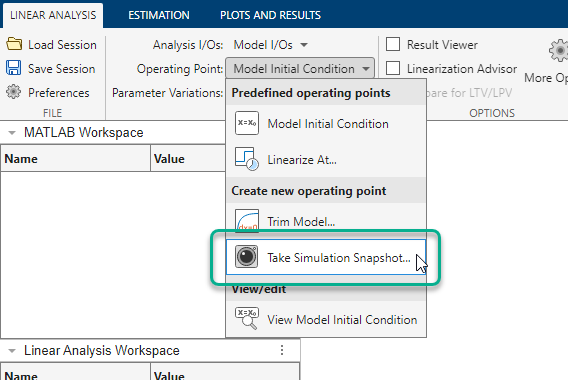In the Enter snapshot times to linearize dialog box, in the Simulation snapshot times field, enter `0.045`, which is enough time for the closed-loop system to reach steady state.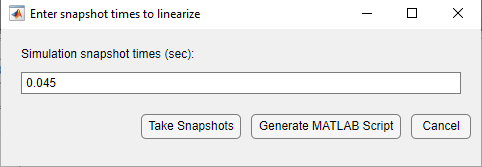Click .

The software simulates the model and creates an operating point that contains the input and state values of the model at the specified snapshot time. This operating point, `op_snapshot1`, is added to the Linear Analysis Workspace.To initialize the model with the computed operating point, double-click `op_snapshot1`.In the Edit dialog box, click .In the Initialize Model dialog box, select MATLAB Workspace, and click . The software exports the operating point to the MATLAB® workspace and initializes the model with the inputs and states in the operating point.

### Specify Controller Structure

Before tuning a PID controller block using PID Tuner, you must specify your controller structure. To do so, double-click the controller block. Then, specify the following controller parameters:

• Controller

• Form

• Time domain

• Discrete-time settings

• Other settings such as the controller initial conditions, output saturation levels, and anti-windup configuration.

For this example, use the current controller configuration; that is, a discrete-time parallel-form PID controller without anti-windup.

Using PID Tuner, you can tune the parameters of the following controller blocks:

If your model uses the Simscape Electrical Discrete PI Controller (Simscape Electrical) block or Discrete PI Controller with Integral Anti-Windup (Simscape Electrical) block, you must replace this block with a Discrete PID Controller block before tuning.

### Identify Plant Model

To open the PID Tuner, click . When PID Tuner first opens, it attempts to linearize the model. Due to the PWM components, the model analytically linearizes to zero.

To obtain a linear plant model, on the PID Tuner tab, click Plant, and then under Create a New Plant, click Identify New Plant.

To identify a plant model, first obtain input/output data by simulating your model. On the Plant Identification tab, click Get I/O Data > Simulate Data. For plant identification, you must specify a finite value for the Simulink model stop time.

On the Simulate I/O Data tab, configure the input signal with the following settings.

• Signal Type of `Step`.

• Sample Time of `5e-06`.

• Onset Lag of `0.025`, which is enough time for the plant to reach steady state.

• Stop Time of `0.07`, which is enough time for the plant output to return to steady state after the step input.

• Offset of `0.736`, which is the value of the PID Controller block output at the computed operating point. For this model, the offset corresponds to the value of the state in the Computational delay block. If you do not have such a corresponding state in your model, you can attach a scope to the output of the PID Controller block and simulate the model at the computed operating point.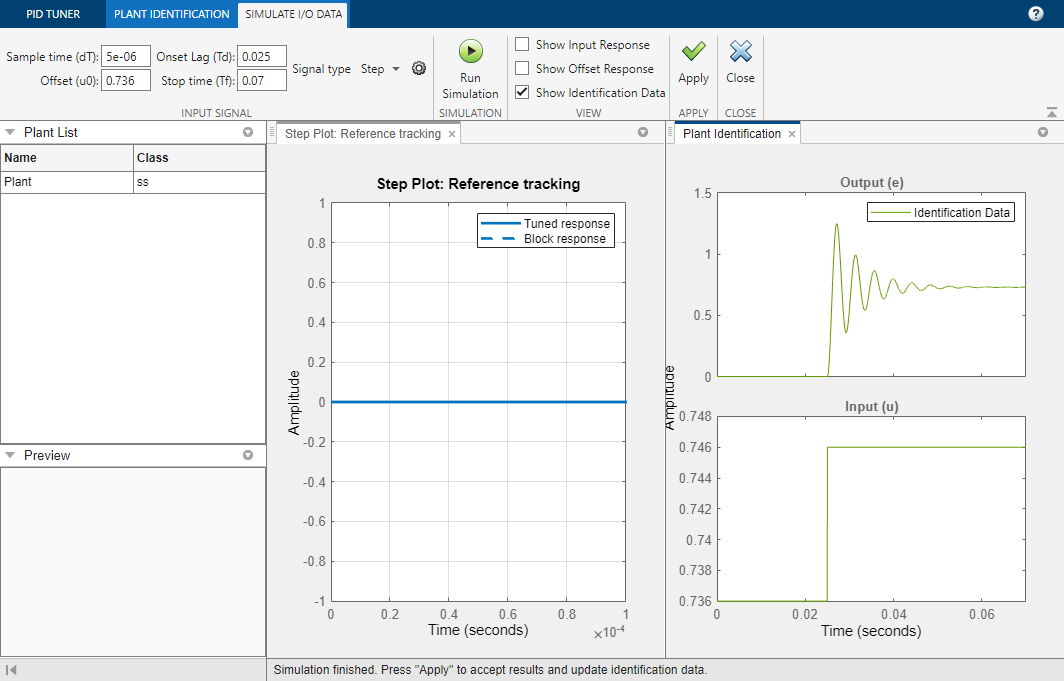To specify the step amplitude, click. Then, in the Step Input Specifications dialog box, in the Amplitude field, type `0.01`. This value is large enough to sufficiently excite the system and small enough to prevent the controller from entering discontinuous-current mode.

Click Run Simulation. To obtain the input/output response of the plant, PID Tuner injects the specified input signal at the output of the PID Controller block and measures the corresponding output response at the input of the controller. The software runs two simulations, an offset response without the input signal and an input response with the input signal. The difference between these responses is the output response.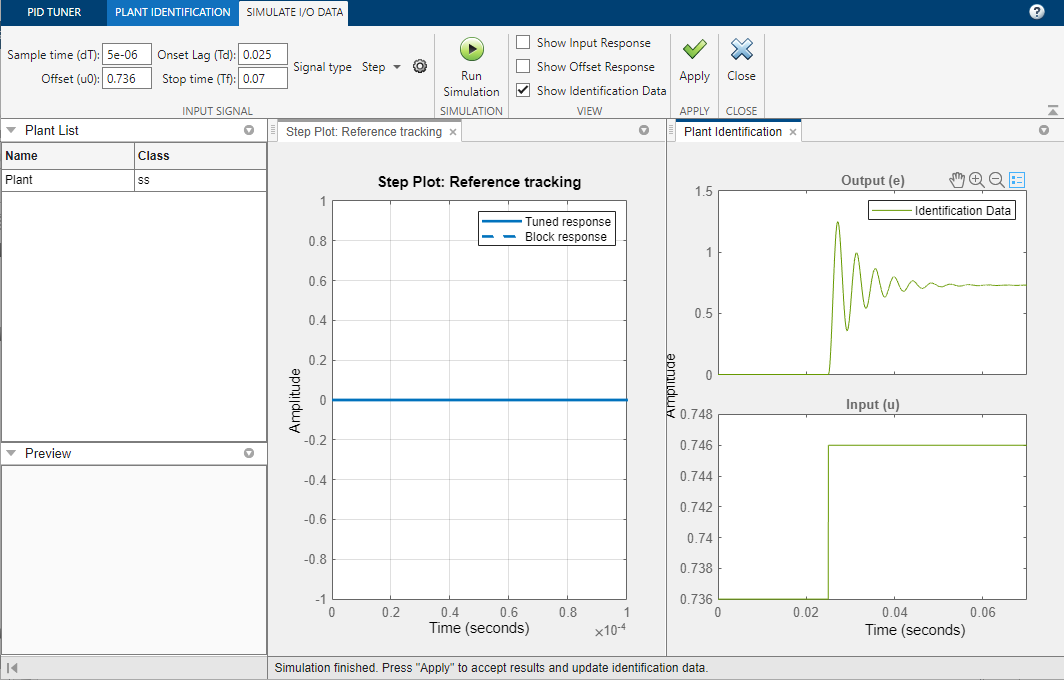In the Plant Identification figure, the Input plot shows the specified input signal, and the Output plot shows the corresponding output response.

To use this simulated input/output data, click Apply. Then, to close the Simulate I/O Data tab, click Close.

On the Plant Identification tab, select the plant structure to identify based on your knowledge of the plant and the appearance of the output step response. For this example, the output response looks like an underdamped second-order response. In the Structure drop-down list, select Underdamped Pair.To obtain a rough approximation of the identified plant, in the Identified Plant Structure plot, drag the dashed lines that correspond to the envelope of the step response. Adjust the response so that it approximates the output response.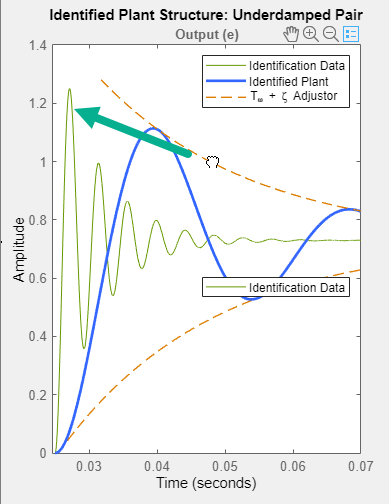To fine-tune the approximate response, click Auto Estimate. The software estimates the parameters of the identified plant model using the current parameters as an initial guess.

The Plant Identification Progress dialog box shows the results of the estimation process. For this example, the fit to the estimation data is greater than 98%. To use this identified plant, on the Plant Identification tab, click Apply.

The PID Tuner updates its identified plant model, selects controller parameters to meet the tuning requirements in the Tuning Tools section, and plots the tuned response of this controller. To expand the plot, close the Plant Identification figure.The step response shows a block response (dashed line) and a tuned response (solid line). The block response corresponds to the current PID gains in the PID Controller block. The tuned response corresponds to the tuned PID gains in PID Tuner.

### Tune Controller

To tune the controller based on bandwidth and phase margin, on the PID Tuner tab, in the Domain drop-down list, select Frequency.

For this example, set the Bandwidth and Phase Margin to `9425` rad/s (1.5 kHz) and `60` deg, respectively, according to the design criteria specified in .

PID Tuner selects controller parameters that meet these design specifications.

To view the frequency response of the tuned system, click Add Plot, and under Bode, click Open-loop.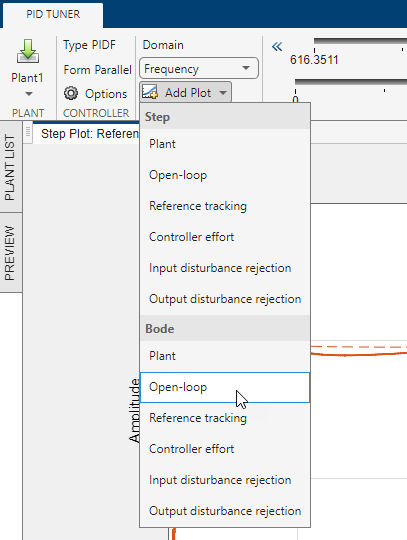To adjust the limits of the Bode plot, right-click the plot area, and select Properties. Then, in the Property Editor dialog box, on the Limits tab, set the axis limits.To view the tuned controller parameters and performance metrics, including the gain and phase margins, click Show Parameters. The tuned result has a 32.7 dB gain margin and 69 deg phase margin at about 9425 rad/s.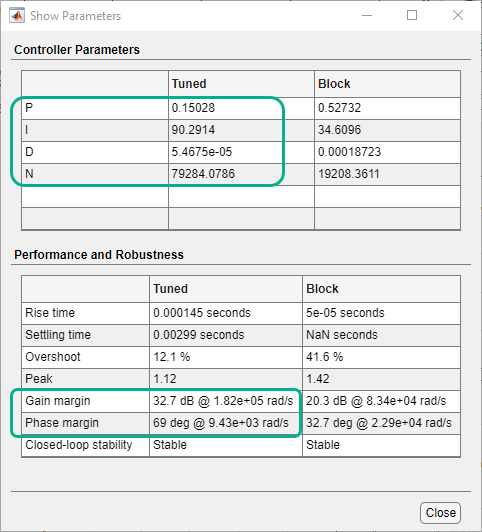To update the PID Controller block with the tuned gains, on the PID Tuner tab, click Update Block.

### Validate Controller

You can examine the tuned controller performance using a simulation with line and load disturbances. To examine the controller dynamic performance, the Simulink model uses the following disturbances:

• Line disturbance at t = 0.075 sec, which increases the input voltage, Vin, from 5V to 10V

• Load disturbance at t = 0.1 sec, which increases the load resistance, Rload, from 3 ohms to 6 ohms

Simulate the model.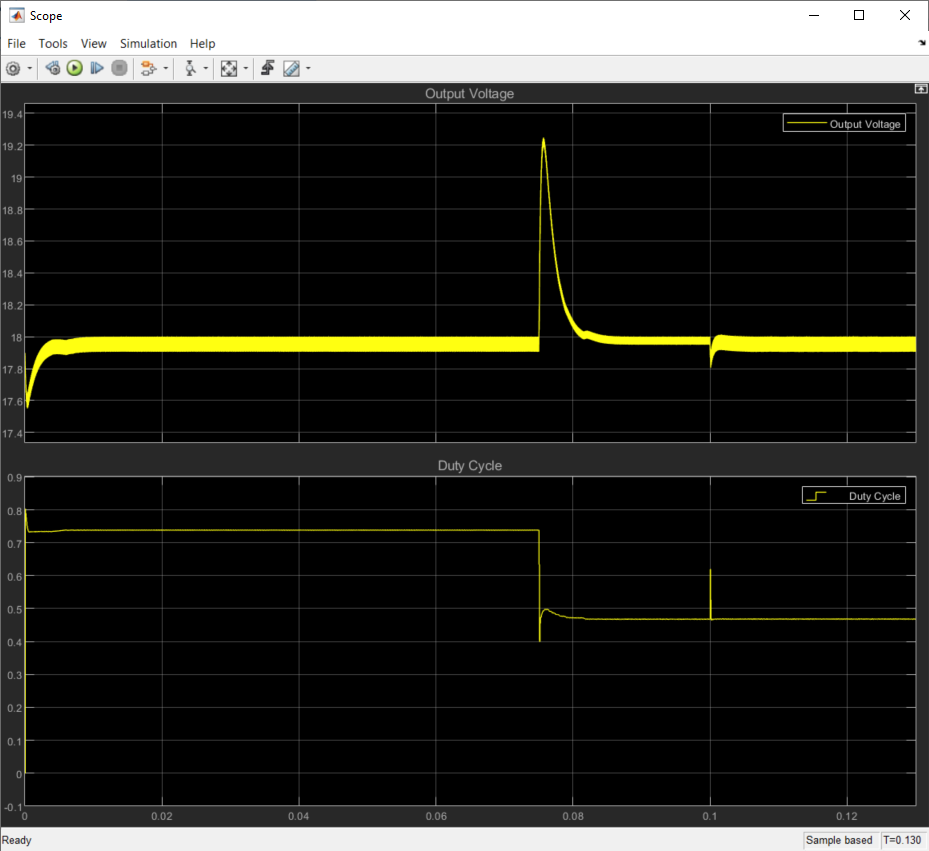The controller rejects the line and load disturbances well.

 Lee, S. W. "Practical Feedback Loop Analysis for Voltage-Mode Boost Converter." Application Report No. SLVA057. Texas Instruments. January 2014. www.ti.com/lit/an/slva633/slva633.pdf